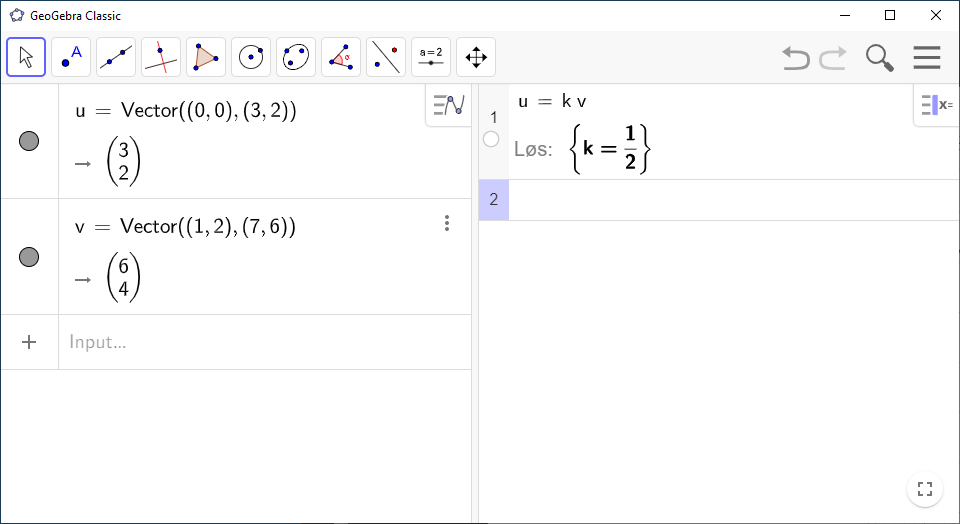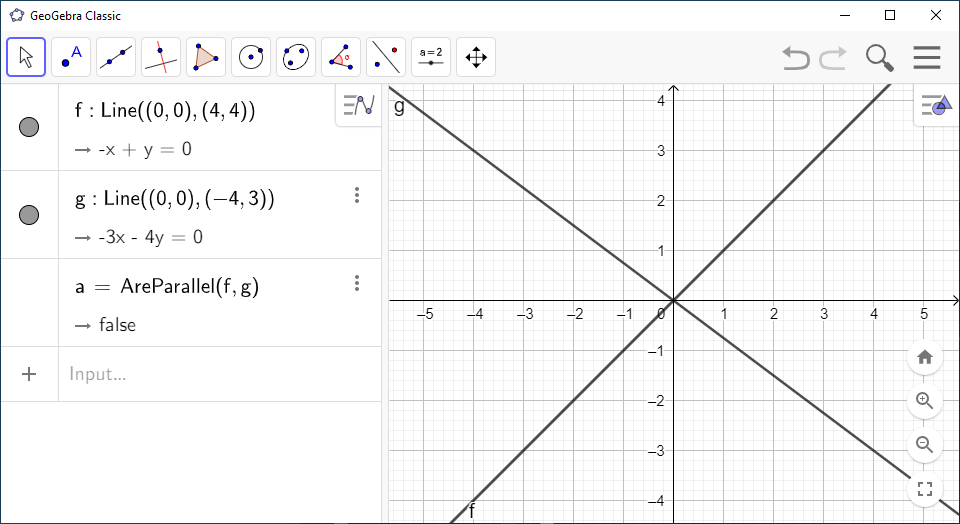# How to Check If Two Vectors are Parallel Using GeoGebra

There are two ways to find out if two vectors are parallel in `GeoGebra`. One is simply to solve the vector equation $\stackrel{\to }{a}=k\cdot \stackrel{\to }{b}$ in `CAS`. The other is to draw two lines and use a `GeoGebra` command to check if the they are parallel.

`GeoGebra` Instruction 1

### Method1

1.
Open `Algebra View` and `Graphics View` under`View` in`Menu`.
2.
Enter your two vectors in `Algebra View`.
3.
Enter

First vector = k * second vector

in `CAS`. Be sure to fill in your vectors with the names they are given in `Algebra View`. Click `Solve`.

4.
If you get a solution for $k$, the vectors are parallel. If you get $\left\{\right\}$, the vectors are not parallel.`GeoGebra` Instruction 2

### Method2

1.
Open `Algebra View` and `Graphics View` in`View` menu.
2.
Define your two vectors in `Algebra View`.
3.
Enter

Line(<Point>, <Direction Vector>)

in `Input Bar`. Fill in `(0,0)` for `<Point>` and one of the vectors for `<Direction Vector>`. Press `Enter`. The line should now be shown `Algebra View` and `Graphics View`.

4.
Repeat the above method for your other vector as well.
5.
Enter

AreParallel(<Line>, <Line>)

in `Algebra View`. Fill in your two lines and press `Enter`. If `true` is displayed in `Algebra View`, the vectors are parallel. Ff `false` is displayed in `Algebra View`, the vectors are not parallel.